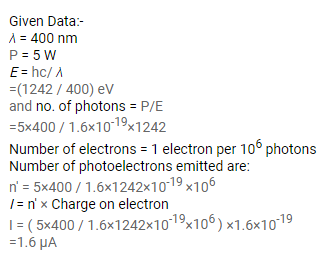# In an experiment on photoelectric effect, light of wavelength

Question:

In an experiment on photoelectric effect, light of wavelength $400 \mathrm{~nm}$ is incident on a cesium plate at the rate of $5.0 \mathrm{~W}$. The potential of the collector plate is made sufficiently positive with respect to the emitter, so that the current reaches its saturation value. Assuming that on average, one out of every $10^{6}$ photons is able to eject a photoelectron, find the photocurrent in the circuit.

Solution: